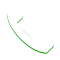0

# What battery should I use for a 1000VA inverter?

Your inverter is rated for a particular (e.g., 12V) DC voltage input. Once you have the DC input voltage, you can divide the power output (VA) of the inverter by the input voltage, to get an idea of the approximate input current that flows from the battery to the inverter, when the inverter is supplying full (1000VA) power to the AC load. If your inverter requires a different DC input voltage, then obviously the DC input current the inverter requires when supplying full power to the associated AC load, will be different. For example, a 12V inverter will draw approximately 1000/12, or about 83 amperes from the battery when at full (1000VA) load. If you multiply that value of current t by the time (in hours) that you want the battery to maintain the inverter load, you will get the approximate ampere-hour (A-h) capacity of the battery you need to supply the inverter. Thus if you want the battery to power the inverter for 2 hours while the latter is supplying 1000VA (I’m going to assume unity power factor, or 1000W) to its associated AC load, you need a battery (or battery bank) of at least 2 x 83.3 = 167 ampere-hours (A-h) capacity.For COD  Orders Call Us